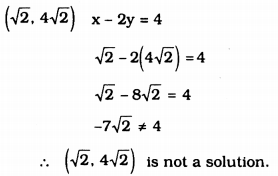# KSEEB Solutions for Class 9 Maths Chapter 10 Linear Equations in Two Variables Ex 10.2

In this chapter, we provide KSEEB SSLC Class 9 Maths Chapter 10 Linear Equations in Two Variables Ex 10.2 for English medium students, Which will very helpful for every student in their exams. Students can download the latest KSEEB SSLC Class 9 Maths Chapter 10 Linear Equations in Two Variables Ex 10.2 pdf, free KSEEB SSLC Class 9 Maths Chapter 10 Linear Equations in Two Variables Ex 10.2 pdf download. Now you will get step by step solution to each question.

## Karnataka Board Class 9 Maths Chapter 10 Linear Equations in Two Variables Ex 10.2

Question 1.
Which one of the following options is true, and why ?
y = 3x + 5 has
i) a unique solution,
ii) only two solutions,
iii) infinitely many solutions.
Solution:
y = 3x + 5 has many solutions. Because this is a linear equation with two variables.

Question 2.
Write four solutions for each of the following equations :
i) 2x + y = 7
ii) πx + y = 9
iii) x = 4y
Solution:
i) 2x + y = 7
If x = 0, 2x + y = 7
2 × 0 + y = 7
0 + y = 7
y = 7
Solution is (0, 7).
If x = 1, 2x + y = 7
2 × 1 + y = 7
2 + y = 7
y = 7 – 2 = 5
Solution is (1, 5).
If x = 2, 2x + y = 7
2 × 2 + y = 7
4 + y = 7
y = 7 – 4 = 3
Solution is (2, 3).
If x = 3, 2x + y = 7
2 × 3 + y = 7
6 + y = 7
∴ y = 7 – 6 = 1
Solution is (3, 1).

ii) πx + y = 9
If x = 0, πx + y = 9
π × 0 + y = 9
0 + y = 9
y = 9
Solution (x, y) = (0, 0).
If x = 1, πx + y = 9
π × 1 + y = 9
π + y = 9
y = 9 – π
Solution (x, y) = (1, 9 – π).
If y = 0, πx + y = 9
πx + 0 = 9
πx = 9
∴ x = 9π
Solution (x, y) = ( 9π, o).
If y = 1, πx + y = 9
πx + 1 = 9
πx = 9 – 1
πx = 8
∴ x = 8π
Solution (x, y) = (8π ,1).

iii) x = 4y
If x = 0, x = 4y
0 = 4y
4y = 0
∴ y = 04 = ∞
∴ Solution (x, y) = (0, ∞).
If x = 1, x = 4y
1 = 4y
4y = 1
∴ y = 14
∴ Solution (x, y) = (1, 14).
If x = 2, x = 4y
2 = 4y
4y = 2
∴ y = 42
∴ y = 12
Solution (x, y) = (2, 12).
If x = 4, x = 4y
4 = 4y
4y = 4
∴ y = 44
∴ y = 1
∴ Solution (x, y) = (4, 1).

Question 3.
Check which of the following are solutions of the equation x – 2y = 4 and which are not:
i) (0, 2)
ii) (2, 0)
iii) (4, 0)
iv) (2–√,42–√)
v) (1, 1)
Solution:
i) (0, 2)
x – 2y = 4
0 – 2(2) = 4
0 – 4 = 4
-4 ≠ 4
∴ (0, 2) is not a solution.

ii) (2, 0)
x – 2y = 4
2 – 2(0) = 4
2 – 0 = 4
2 ≠ 4
∴ (2, 0) is not a solution

iii) (4, 0)
x – 2y = 4
4 – 2(0) = 4
4 – 0 = 4
4 = 4
∴ (4, 0) is a solution.

iv)v) (1, 1)
x – 2y = 4
1 – 2(1) = 4
1 – 2 = 4
-1 ≠ 4
∴ (1, 1) is not a solution.

Question 4.
Find the value of k, if x = 2, y = 1 is a solution of the equation 2x + 3y = k.
Solution:
2x + 3y = k
If x = 2, y = 1, then k = ?
2x + 3y = k
2(2) + 3(1) = k
4 + 3 = k
7 = k
∴ k = 7.

All Chapter KSEEB Solutions For Class 9 Maths

—————————————————————————–

All Subject KSEEB Solutions For Class 9

*************************************************

I think you got complete solutions for this chapter. If You have any queries regarding this chapter, please comment on the below section our subject teacher will answer you. We tried our best to give complete solutions so you got good marks in your exam.

If these solutions have helped you, you can also share kseebsolutionsfor.com to your friends.

Best of Luck!!